# Autoencoders and latent space fragmentation – V – reconstruction of human face images from simple statistical z-point-distributions?

In this post series we have so far studied the distribution of latent vectors and z-points for CelebA images in the latent space of an Autoencoder [AE]. The CelebA images show human faces. We want to reconstruct images with new faces from artificially created, statistical latent vectors. Our latent space had a number dimensions N=256. For basics of Autoencoders, terms like latent space, z-points, latent vectors etc. see the first blog of this series:

Autoencoders and latent space fragmentation – I – Encoder, Decoder, latent space

During the experiments and analysis discussed in the other posts

we have learned that the multi-dimensional latent space volume which the Encoder of a convolution AE fills densely with z-points for CelebA images has a special shape and location. The bulk of z-points is confined to a multi-dimensional ellipsoid which is relatively small. Its center has a position, which does not coincide with the latent space’s origin. The bulk center is located within or very close to a hyper-sub-volume spanned by only a few coordinate axes.

We also saw also that we have difficulties to hit this region by artificially created z-points via latent vectors. Especially, if the approach for statistical vector generation is a simple one. In the beginning we had naively assumed that we can treat the vector components as independent variables. We assigned each vector component values, which we took from a value-interval [-b, b] with a constant probability density. But we saw that such a simple and seemingly well founded method for statistical vector creation has a variety of disadvantages with respect to the bulk distribution of latent vectors for CelebA images. To name a few problems:

• The components of the latent vectors for CelebA images are correlated and not independent. See the third post for details.
• If we choose b too large (&gt. 2.0) then the length or radius values of the created vectors will not fit typical radii of the CelebA vectors. See the 2nd post for details.
• The generated vectors only correspond to z-points which fill parts of a thin multi-dimensional spherical shell within the cube filled by points with coordinate values -b < x_j < +b. This is due to mathematical properties of the distribution in multi-dimensional spaces. See the second post for more details.
• Optimal parameter values are 1.0 < b < 2.0, just to guarantee at least the right vector length. However, due to the fact that the origin of the latent space is located in a border region of the CelebA bulk z-point distribution, most points will end up outside the bulk volume.

In this post I will show you that our statistical vectors and the related z-points do indeed not lead to a successful reconstruction of images with clearly visible human faces. Afterward I will briefly discuss whether we still can have some hope to create new human face images by a standard AE’s Decoder and statistical points in its latent space.

# Relevant values of parameter b for the interval from which we choose statistical values for the vector components

What does the number distribution for the length of latent CelebA vectors look like? For case I explained in the 2nd post of this series we get: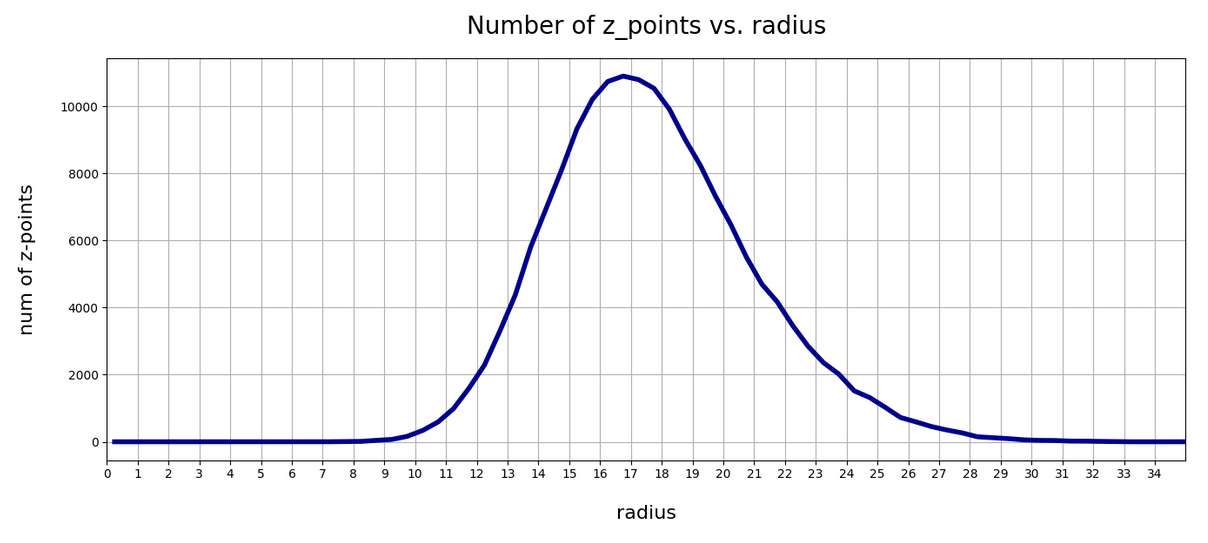For case II: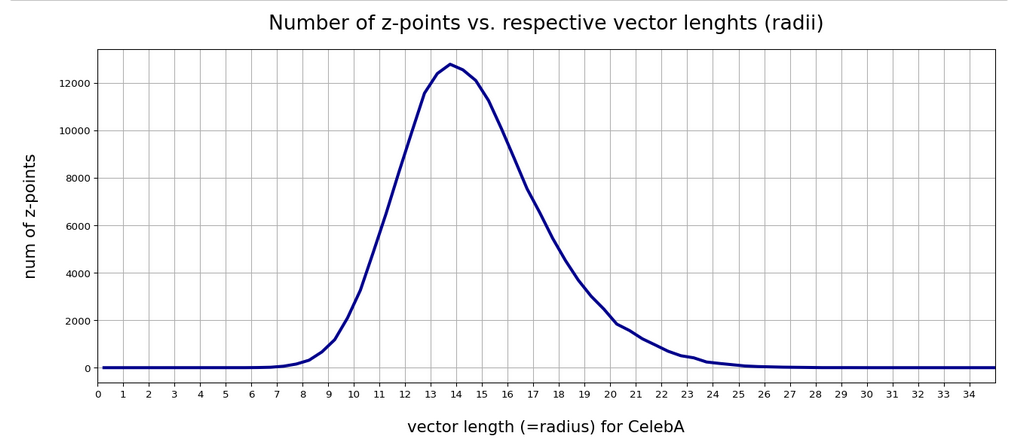Now let me remind you of a comparison with the lengths of statistical vectors for different values of b: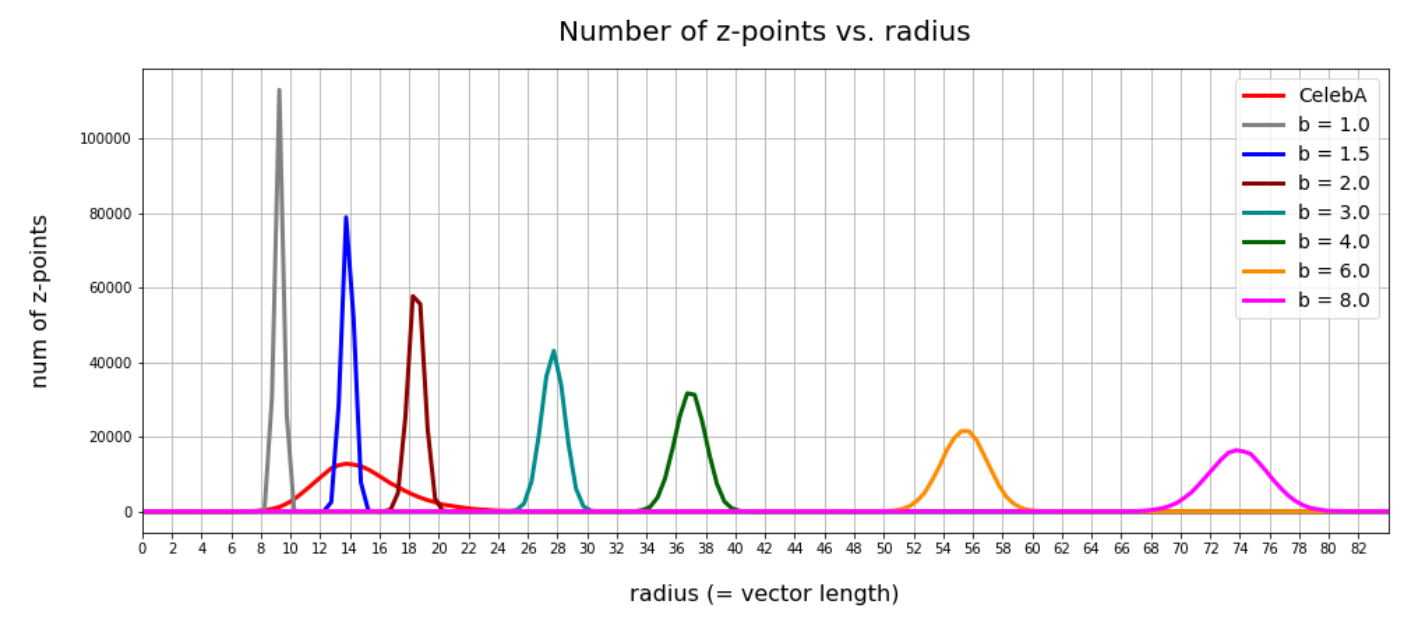See the 2nd post of this series for more details. Obviously, for our simple generation method used to create statistical vectors a parameter value b = 1.5 is optimal. b=1.0 an b=2.0 mark the edges of a reasonable range of b-values. Larger or smaller values will drive the vector lengths out of the required range.

# Image reconstructions for statistical vectors with independent component values x_j in the range -1.5 < x_j < 1.5

I created multiples of 512 images by feeding 512 statistical vectors into the Decoder of our convolutional Autoencoder which was trained on CelebA images (see the 1st and the 2nd post for details). The vector components x_j fulfilled the condition :

-1.5 ≤   x_j   ≤ 1.5,    for all j in [0, 256]

The result came out to be frustrating every time. Only singular image showed like outlines of a human face. The following plot is one example out of series of tenths of failures.

And this was an optimal b-value! 🙁 But due to the analysis of the 4th post in this series we had to expect this.

# Image reconstructions for statistical vectors with independent component values x_j in the range -1.0 < x_j < 1.0

The same for

-1.0 ≤   x_j   ≤ 1.0,    for all j in [0, 256]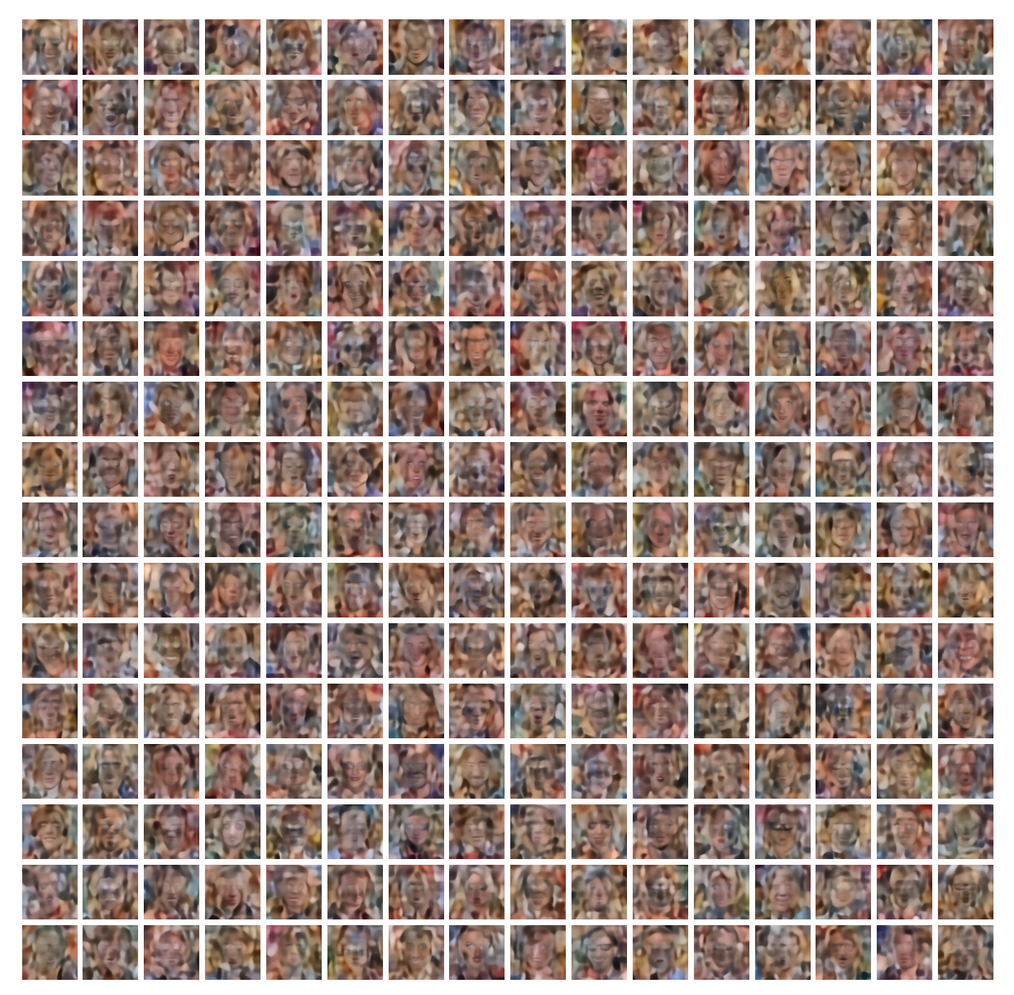# Image reconstructions for statistical vectors with independent component values x_j in the range -2.0 < x_j < 2.0

The same for

-2.0 ≤   x_j   ≤ 2.0,    for all j in [0, 256]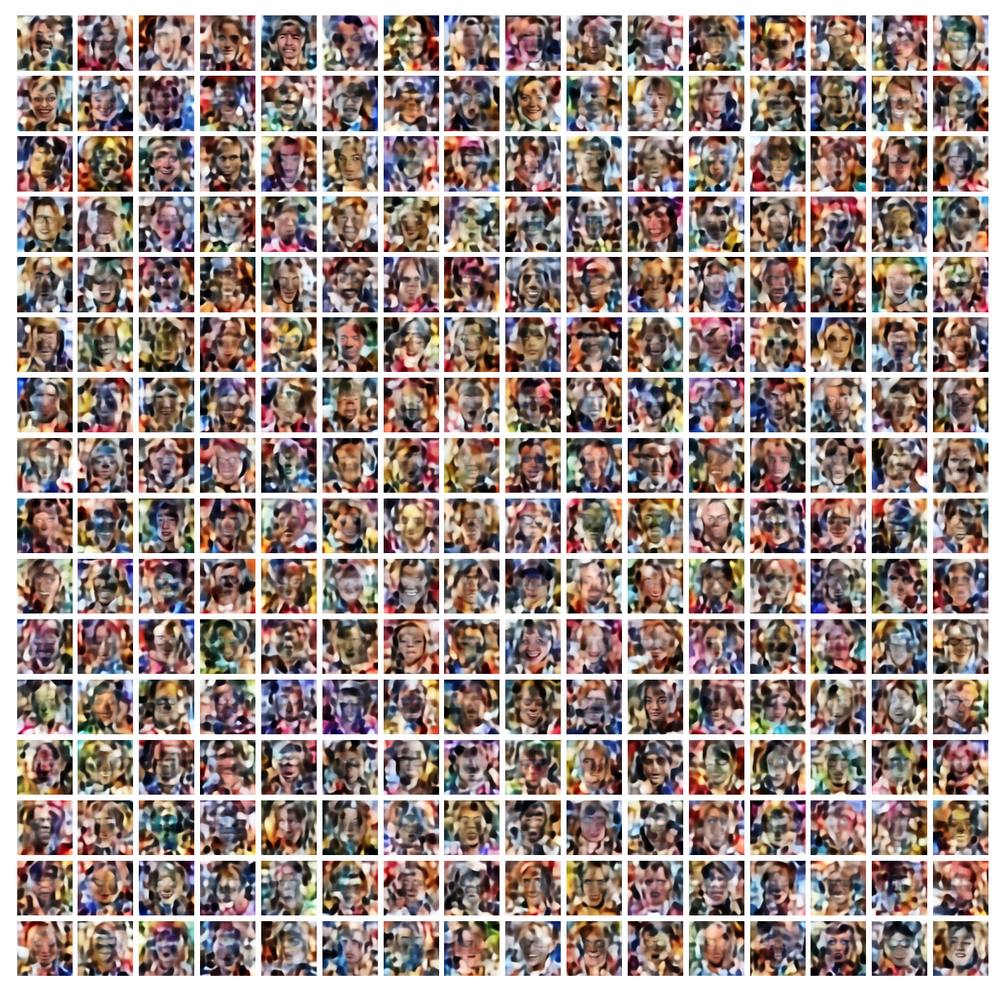# Image reconstructions for statistical vectors with independent component values in the range -5.0 < x_j < 5.0

If you have read the previous posts in this series then you may think: Some of the component values of latent vectors for CelebA images had bigger values anyway. So let us try:

-1.0 ≤   x_j   ≤ 1.0,    for all j in [0, 256]

And we get: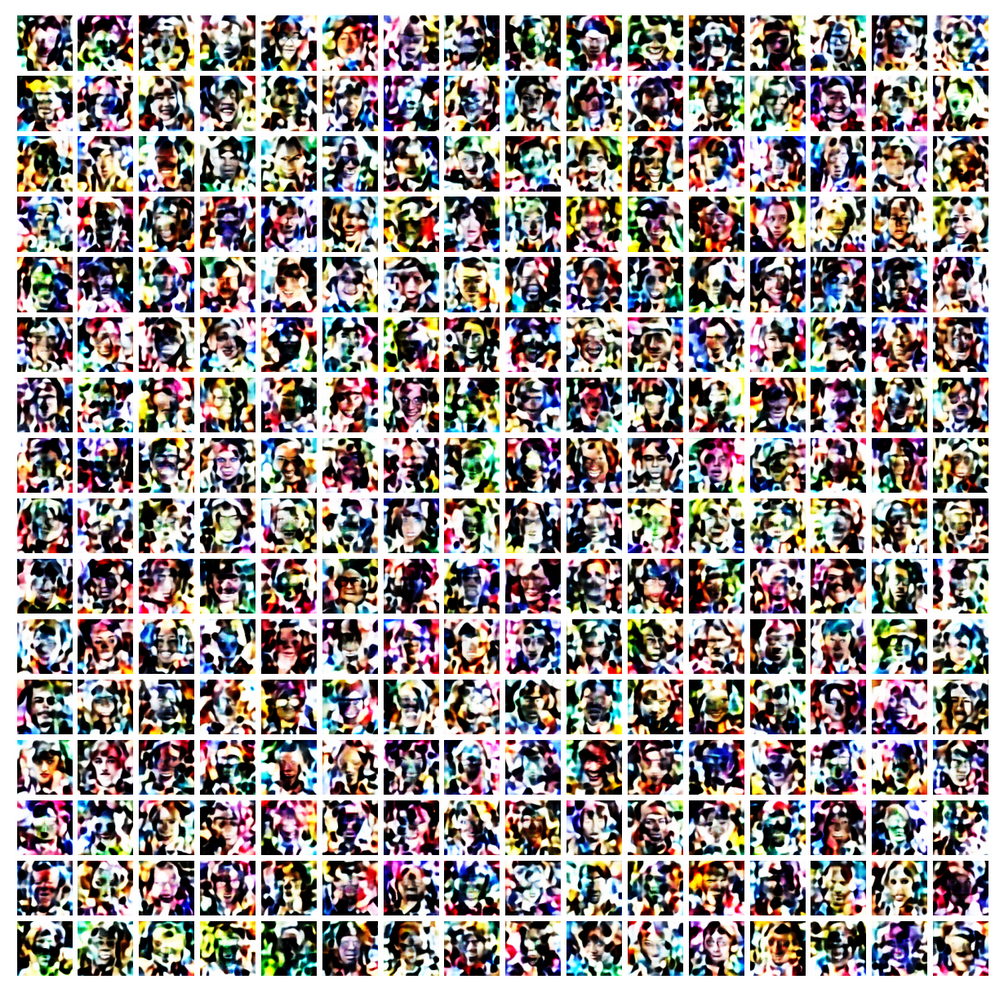Although some component values may fall into the value regions of latent CelebA vectors most of them do not. The lengths (or radii) of the statistical vectors for b=5.0 do not at all fit the average radii of latent vectors for CelebA images.

# What should we do?

I have described the failure to create reasonable images from statistical vectors in some regions around the origin of the latent space also in other posts on standard Autoencoders and in posts on Variational Autoencoders [VAEs]. For VAEs one enforces that regions around the origin are filled systematically by special Encoder layers.

But is all lost for a standard Encoder? No, not at all. It is time that we begin to use our knowledge about the latent z-point distribution which our AE creates for CelebA images. As I have shown in the 2nd post it is simple to get the number distribution for the component values. Because these distributions were similar to Gaussians we have rather well defined value regions per component which we can use for vector creation. I.e. we can perform a kind of first order approximation to the main correlations of the components and thus put our artificially created values inside the densely populated bulk of the z-point region for CelebA images. If that region in the latent space has a meaning at all then we should find some interesting reconstruction results for z-points within it.

We can do this even with our simple generation method based on constant probability densities, if we use individual value regions -b_j ≤ x_j ≤ b_j for each component. (Instead of a common value interval for all components). But even better would be Gaussian approximations to the real number distributions for the component values. In any case we have to restrict the values for each component much stronger than before.

# Conclusion

What we already had expected from previous analysis became true. A simple method for the creation of statistical latent vectors does not give us z-points which are good for the creation of reasonable images by a trained AE’s Decoder. The simplifications

1. that we can treat the component values of latent vectors as independent variables
2. and that we can assign the components x_j values from a common interval -b ≤ x_j &le b

cause that we miss the bulk region in the AE’s latent space, which gets filled by its trained Encoder. We have demonstrated this for the case of an AE which had been trained on images of human faces.

In the next post

Autoencoders and latent space fragmentation – VI – image creation from z-points along paths in selected coordinate planes of the latent space

I will show that even a simple vector creation can give us latent vectors within the region filled for CelebA images. And we will see that such points indeed lead to the reconstruction of images with clearly visible human face images by the Decoder of a standard AE trained on the CelebA dataset.